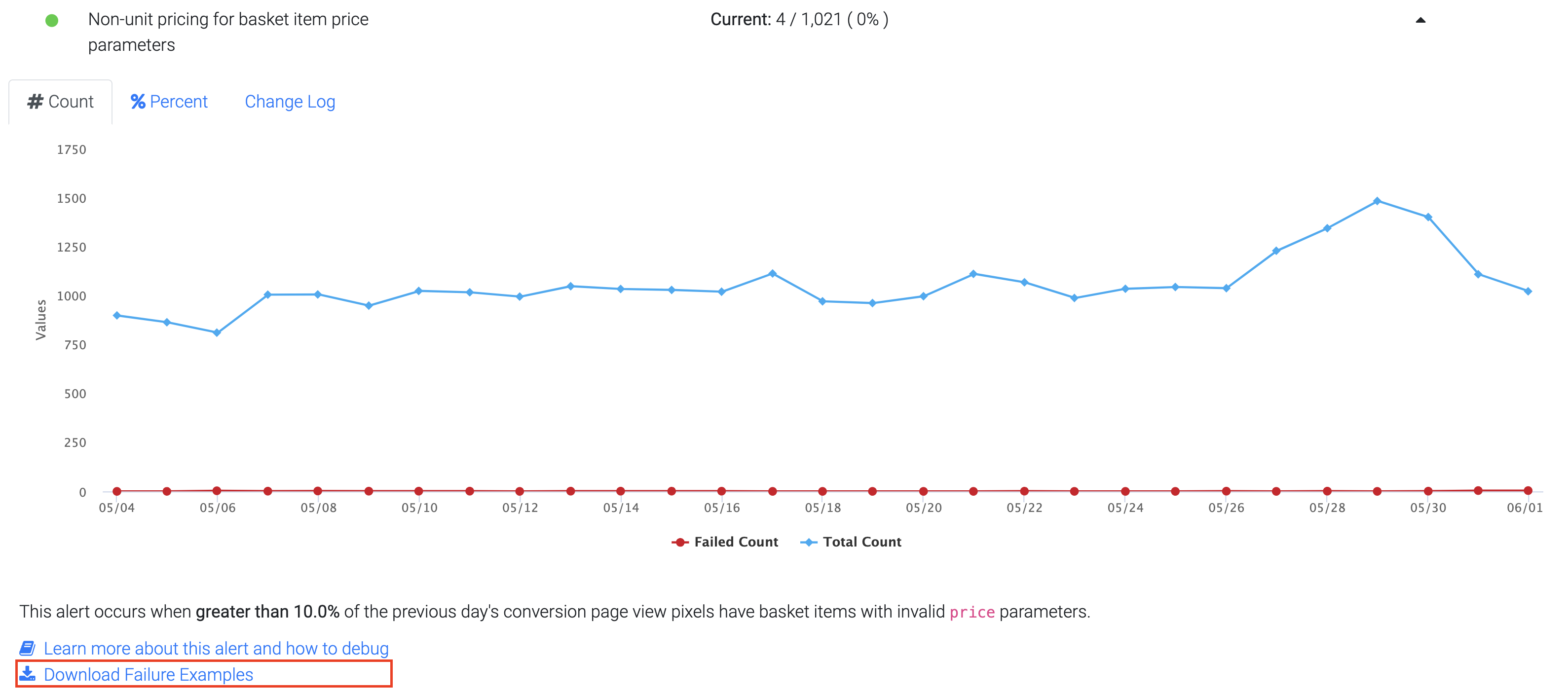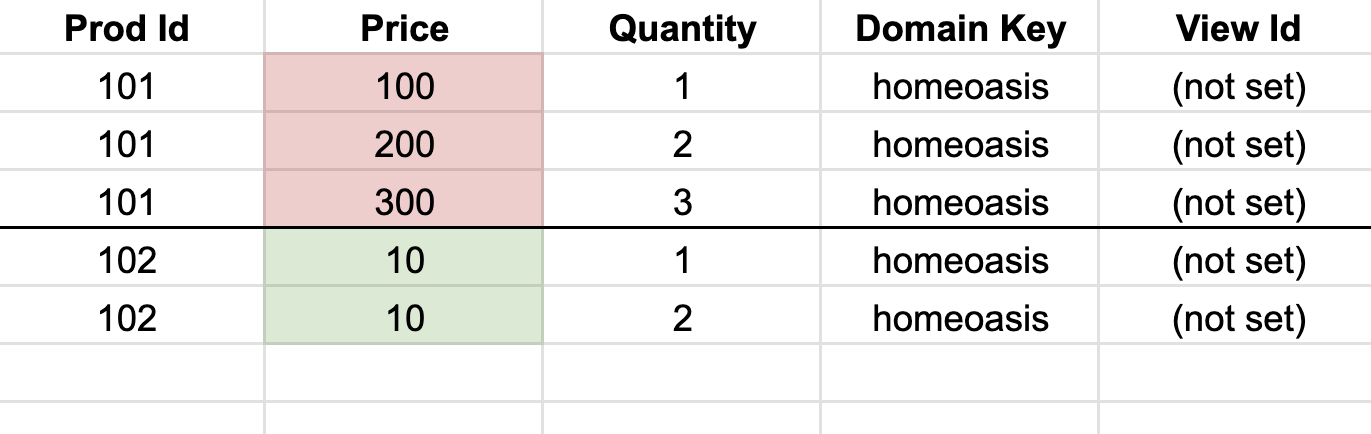# Non-unit pricing for basket item price parameters

## Problem and Background

The Conversion Basket Page View pixel requires each item in the basket parameter to be listed with its price and quantity. The price should be the price of a single unit. Inserting price*quantity in the price field is incorrect. This check tries to catch that case.

For instance, if a shopper buys 2 of an item that is priced at \$10 each:

Correct:

Price: \$10
Quantity: 2

Incorrect:

Price: \$20
Quantity: 2

This check works as follows:

• For a given day, it looks for PIDs that have had purchases of quantity=1 and also of quantity>1.
• Then it takes the [price/quantity] for quantity = 1, 2, … whatever quantity values are present.
• If the price value is correct, the [price/quantity] is expected to change at different quantities. If the [price/quantity] value is the same for different quantities, it is flagged as an error.
• The final percentage reported in the metric is calculated as{# of cases where price/quantity for a given PID is same for different quantities} / {# of cases where a PID is purchased at different quantities}

Example

Let's walk through an example to illustrate each step:

Suppose we have a specific day's sales data for 2 products:

Product ID (PID)QuantityPrice [\$]
PID-1110
PID-1220
PID-2115
PID-2315

Step 1: Identify PIDs with purchases of quantity=1 and quantity>1.

In this example, PIDs "PID-1" and "PID-2" meet this condition.

Step 2: Calculate [price/quantity] for each purchase.

For PID-1:

For quantity = 1: [price/quantity] = \$10 / 1 = \$10

For quantity = 2: [price/quantity] = \$20 / 2 = \$10

For PID-2:

For quantity = 1: [price/quantity] = \$15 / 1 = \$15

For quantity = 3: [price/quantity] = \$15 / 3 = \$5

Step 3: Check if [price/quantity] values change for different quantities.

For PID-1, the [price/quantity] remains the same (\$10) for different quantities. This indicates a bad case.

Step 4: Calculate the final percentage for the metric.

In this example, there are 2 cases where a PID is purchased at different quantities (PID-1 and PID-2). Out of these, 1 case (PID-1) has [price/quantity] that remains the same for different quantities.

Hence, the final percentage reported in the metric would be 1 (the number of cases where [price / quantity] is the same) divided by 2 (the number of cases where a PID is purchased at different quantities), resulting in 50%.

## Debugging Steps

To debug this issue, follow the steps below:• Check if you’re not sending price*quantity in the Price field. You must send the price of the individual product for different quantities.

In the example below, incorrect values (price*quantity) were sent for PID 101 (color-coded in red). Correct values were sent for PID 102 (color-coded in green).## Common Causes

This issue usually occurs when the price field is set as price*quantity instead of just price on your end.

## Solution

Check the issue using the debugging steps and fix the pixel implementation.

👍